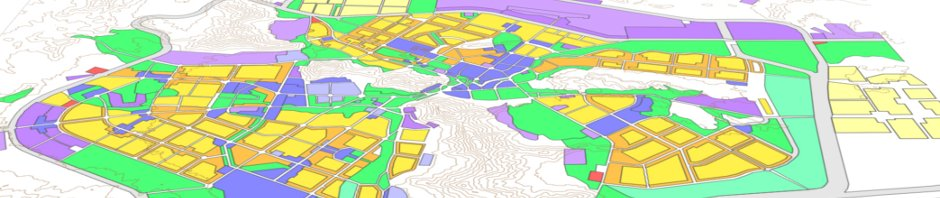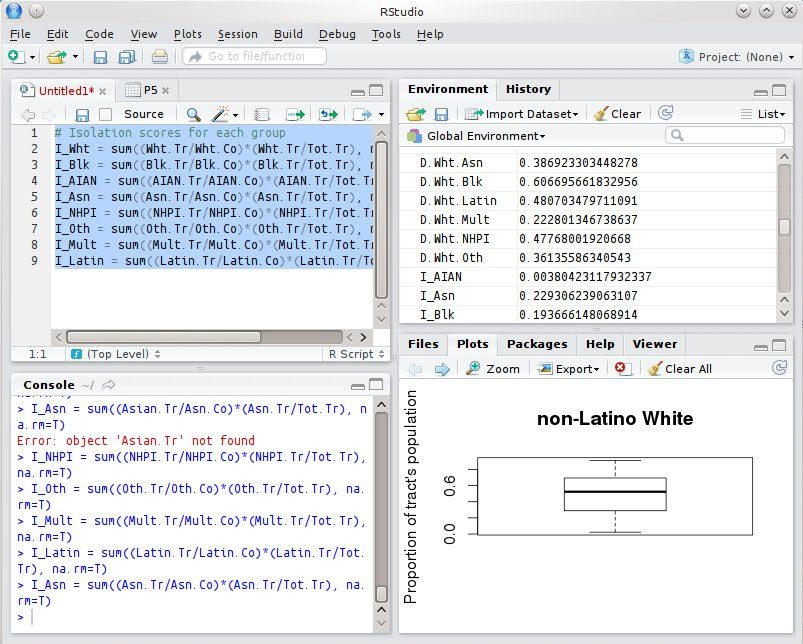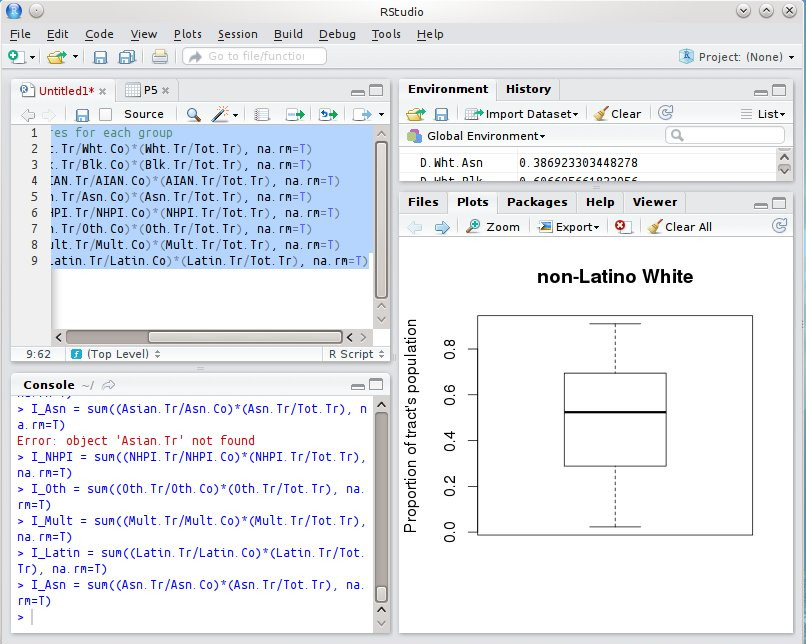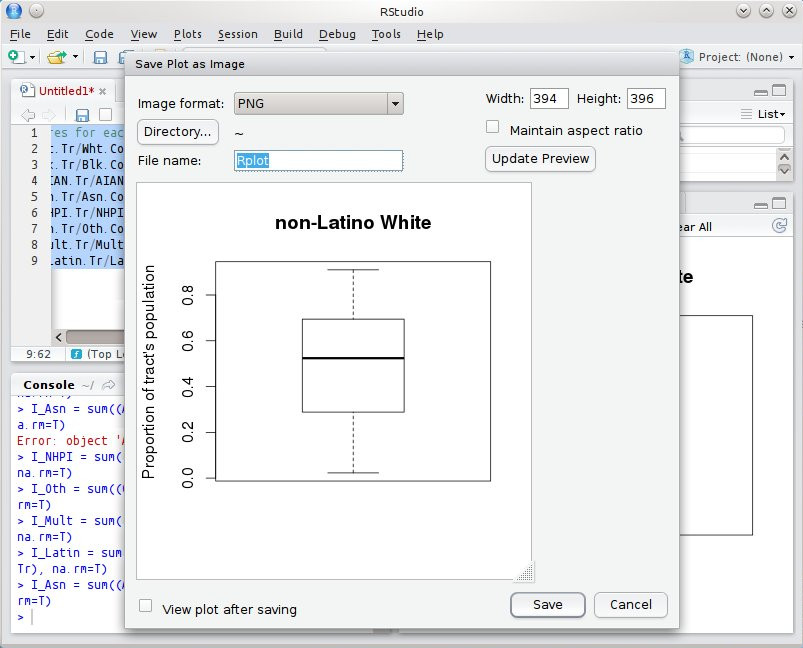# Boxplots and Histograms in R

Dec 21, 2014: variable-names on this page are now consistent with naming conventions.

On the upper part of this page I am adding the updated instructions for generating boxplots and histograms using the newer syntax. In the newer syntax, variable-names don’t have periods in them, and you always need to call out the dataframe (“CO”) so you don’t have to rely on the attach() command.

```# Descriptive graphics for proportion of tract's population comprised of each group
boxplot(CO\$prpWhtTr, main="non-Latino White", ylab="Proportion of tract's population")
hist(CO\$prpWhtTr, ylab = "Number of tracts", xlab = "Proportion", main="Prop. non-Latino White in tracts")
boxplot(CO\$prpBlkTr, main="non-Latino Black", ylab="Proportion of tract's population")
hist(CO\$prpBlkTr, ylab = "Number of tracts", xlab = "Proportion", main="Prop. non-Latino Black in tracts")
boxplot(CO\$prpNtvTr, main="non-Latino AIAN", ylab="Proportion of tract's population")
hist(CO\$prpNtvTr, ylab = "Number of tracts", xlab = "Proportion", main="Prop. non-Latino AIAN in tracts")
boxplot(CO\$prpAsnTr, main="non-Latino Asian", ylab="Proportion of tract's population")
hist(CO\$prpAsnTr, ylab = "Number of tracts", xlab = "Proportion", main="Prop. non-Latino Asian in tracts")
boxplot(CO\$prpApiTr, main="non-Latino NHPI", ylab="Proportion of tract's population")
hist(CO\$prpApiTr, ylab = "Number of tracts", xlab = "Proportion", main="Prop. non-Latino NHPI in tracts")
boxplot(CO\$prpOthTr, main="non-Latino some other race", ylab="Proportion of tract's population")
hist(CO\$prpOthTr, ylab = "Number of tracts", xlab = "Proportion", main="Prop. non-Latino other in tracts")
boxplot(CO\$prpMltTr, main="non-Latino multiracial", ylab="Proportion of tract's population")
hist(CO\$prpMltTr, ylab = "Number of tracts", xlab = "Proportion", main="Prop. non-Latino multiracial in tracts")
boxplot(CO\$prpLatTr, main="Latino", ylab="Proportion of tract's population")
hist(CO\$prpLatTr, ylab = "Number of tracts", xlab = "Proportion", main="Prop. Latino in tracts")```

Sometimes I have run this command and R Studio has returned an error; I think because it decides that the lower right-hand View Pane is too small to fit the generated graphics. I’m not sure. Even if it succeeds, the graphic output may look “squished” in the default four-pane layout:However you can stretch and shift the size of the panes in R, so I did this:At this point, you can click the “Export” button on the top of the View Pane and export the graphic. You can save it as a PDF, but I recommend saving as a PNG. PNG is a “raster” file format. [What is a “raster image”, you ask? Well, most digital images are some sort of raster image: any picture you take with a digital camera, any scan, these are all rasters. They can be saved in various file formats, like TIFF, GIF, JPEG, and PNG. For our purposes, PNG is the best overall format] Once you have saved the graphic as a PNG image-file, you can Insert-Image into a Word document, such as your term paper.In the highlighted-blue field in the image above, I recommend naming the file something sensible, like WhtPropTract_BoxPlot.png.

In that same “View” Pane in R Studio, just below the File Menu button, are left and right arrows. Using those arrows, you can scroll through the 8 histograms and 8 boxplots you have just created. Export and save each of them as a PNG image-file with a filename that is sensible to you. Then, paste them all into a Word document either in MS Word or LibreOffice Writer. Save the file (as a .docx or .odt), and then you can upload it to iLearn and also use that document as part of your term paper.